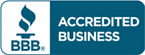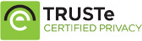Sale!

# Solution manual for Introduction to Econometrics 3rd edition by James H. Stock, Mark W. Watson

\$47.00 \$30.00

Introduction to Econometrics 3rd edition by James H. Stock, Mark W. Watson Answers for Questions and Exercises

Full Chapters are included

Files include :

• Solutions to End-of-Chapter Empirical Exercises
• Solutions to End-of-Chapter Exercises

Do you need Test bank for this book?## Description

Solution manual for Introduction to Econometrics 3rd edition by James H. Stock, Mark W. Watson

Part I. Introduction and Review
Chapter 1. Economic Questions and Data
Chapter 2. Review of Probability
Chapter 3. Review of Statistics
Part II. Fundamentals of Regression Analysis
Chapter 4. Linear Regression with One Regressor
Chapter 5. Regression with a Single Regressor: Hypothesis Tests and Confidence Intervals
Chapter 6. Linear Regression with Multiple Regressors
Chapter 7. Hypothesis Tests and Confidence Intervals in Multiple Regression
Chapter 8. Nonlinear Regression Functions
Chapter 9. Assessing Studies Based on Multiple Regression
Part III. Further Topics in Regression Analysis
Chapter 10. Regression with Panel Data
Chapter 11. Regression with a Binary Dependent Variable
Chapter 12. Instrumental Variables Regression
Chapter 13. Experiments and Quasi-Experiments
Part IV. Regression Analysis of Economic Time Series Data
Chapter 14. Introduction to Time Series Regression and Forecasting
Chapter 15. Estimation of Dynamic Causal Effects
Chapter 16. Additional Topics in Time Series Regression
Part V. The Econometric Theory of Regression Analysis
Chapter 17. The Theory of Linear Regression with One Regressor
Chapter 18. The Theory of Multiple Regression